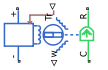# Motor & Drive

Generic motor and drive with closed-loop torque control

Since R2021a

•Libraries:
Simscape / Driveline / Engines & Motors

## Description

The Motor & Drive block represents a generic brushless motor and drive with closed-loop torque control. It is a simplified version of the Motor & Drive (System Level) (Simscape Electrical) block. The Motor & Drive block is useful if you need a generic or low-fidelity motor implementation in your system. It is also suited for cases when you do not know all of your motor specifications or you want to use the block to find an appropriate motor for your system.

To enable faster simulation, the block abstracts the motor, drive electronics, and control. The block generates a torque-speed envelope that saturates the input torque, and it permits only the range of torques and speeds that the envelope defines.

### Modeling Electrical Losses

The Motor & Drive block models first-order losses based on the overall efficiency for a given speed and torque, which you specify as Motor and driver overall efficiency (percent), Speed at which efficiency is measured, and Torque at which efficiency is measured, respectively. The block uses the speed and torque to generate a torque-speed envelope. The envelope saturates the input torque, which yields the torque that the motor responds to, τelec. This is also the torque that the block uses to compute the electrical losses.

The block only considers torque-dependent resistive losses such that

`${P}_{losses}=k{\tau }_{elec}^{2},$`

where

`$k=\frac{{\omega }_{\eta }\left(1-\eta /100\right)}{{\tau }_{\eta }\cdot \eta /100}.$`

Resistive losses are also known as Ohmic losses and occur due to the tendency of the armature windings to resist the flow of electrons. The electrical power includes these losses such that

`${P}_{elec}={P}_{losses}+\omega {\tau }_{elec}.$`

The rate of conversion from electrical energy to heat energy is defined by Joule's law:

`$I=\frac{{P}_{elec}}{V},$`

where:

• Pelec is the electrical power that the block calculates and uses in the governing equation.

• Plosses is the electrical power lost during operation. When you model the effects of heat flow and temperature change, this value represents the rate of heat flow that gets distributed into the thermal mass or out port H.

• ω is the angular velocity of the rotor. This is equivalent to the W output port value.

• τelec is the saturated torque demand.

• k is the proportionality constant for resistance losses, which has the units (energy*time)-1.

• η is the efficiency of the motor and driver for a given speed and torque. This value is equivalent to the Motor and driver overall efficiency (percent) parameter.

• ωη is the angular velocity that corresponds to the overall efficiency. This value is equivalent to the Speed at which efficiency is measured parameter.

• τη is the torque that corresponds to the overall efficiency. This value is equivalent to the Torque at which efficiency is measured parameter.

• V is the voltage across the terminals.

• I is the current through the terminals.

When you enable thermal modeling, Plosses represents the contribution from the block to the heat flow.

To include series resistance, fixed losses, and iron losses, you can add blocks to your model or use the Motor & Drive (System Level) (Simscape Electrical) block.

Tip

You can add damping and inertia with the Rotational Damper block and Inertia block, respectively.

### Thermal Modeling

You can model the effects of heat flow and temperature change by enabling the optional thermal port. To enable the port, set Thermal port to `Model`.

When you model the effects of heat flow and temperature change, the electrical losses from the motor contribute to these effects.

### Variables

Use the Variables tab to set the priority and initial target values for the block variables before simulating. For more information, see Set Priority and Initial Target for Block Variables.

Dependencies

To enable this setting, set Thermal port to `Model`.

### Assumptions and Limitations

• The motor driver tracks a torque demand with the time constant Tc.

• Motor speed fluctuations due to mechanical load do not affect the motor torque tracking.

## Ports

### Input

expand all

Physical signal input port associated with the reference torque demand.

### Output

expand all

Physical signal output port associated with the mechanical rotational speed.

### Conserving

expand all

Electrical conserving port associated with the positive electrical DC supply.

Electrical conserving port associated with the negative electrical DC supply.

Mechanical rotational conserving port associated with the motor case.

Mechanical rotational conserving port associated with the motor rotor.

Thermal conserving port associated with heat flow. Electrical losses from the motor contribute to the heat flow through this port.

#### Dependencies

To enable this port, set Thermal port to `Model`.

## Parameters

expand all

Maximum permissible torque value. The block uses this value and the Maximum power parameter to define the torque-speed envelope.

Maximum permissible power value. The block uses this value and the Maximum torque parameter to define the torque-speed envelope.

Torque controller output time interval. Use this parameter to tell the block how long to wait between issuing torque value output information.

Efficiency to convert from electrical to mechanical rotational energy.

Speed that the block uses to calculate torque-dependent electrical losses.

Torque that the block uses to calculate torque-dependent electrical losses.

Option to enable the thermal port and include thermal losses in your simulation.

Thermal mass of the electrical winding, defined as the energy required to raise the temperature by one temperature unit.

#### Dependencies

To enable this parameter, set Thermal port to `Model`.

## Version History

Introduced in R2021a